• 前言
• 二维RGB图像
• 成像原理
• 数据准备
• 图片加载
• 算法实现
• 生成点云
• 点云显示
• 完整代码
• 总结
• # 前言

废话不多说，直接开造。这里的话我们有两个目标，第一个是如何把一个2维图片上的点映射到3维空间。第二就是如何生成3D点云。当然实际上这是一个大问题，因为只要解决了第一个问题，第二个问题就是“送分”

# 二维RGB图像

在说到3D点云之前我们就不得不说到RGB图像，也就是一些二维图像。

图像以像RGB三个通道的形式进行存储。也就是这样：平时我们看到的就是左边的2D图像，实际上是以右边的形式存储的。

那么从我们的矩阵角度来看的话，大概是这个样子的：

``````[
[[r,g,b],[r,g,b],[r,g,b],[r,g,b]],
[[r,g,b],[r,g,b],[r,g,b],[r,g,b]],
...

]
``````

这里面存在了两组信息，
第一组就是我们最容易忽略的位置信息，也就是像素点的位置。
第二组就是我们的色彩信息。

显然如果我们想要生成3D点云的话，我们的色彩信息是必要的。

而位置信息，在二维平面上，是在3为空间上的映射

那么要想得到3D点云，那么我们就必须得到完整的位置坐标，也就是x,y,z

# 成像原理

那么说到这里的话，我们就不得不说到，摄像机是如何把咱们的影像映射出来的了。

大概他是这样成像的：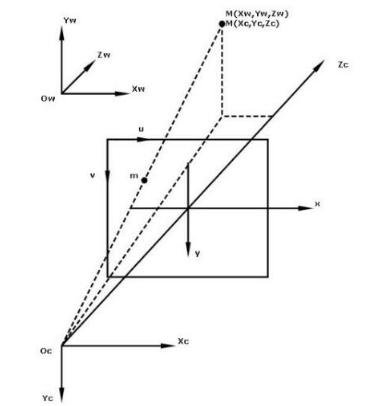这里咱们有三个东西需要注意一下。

首先是我们的摄像机位置
之后是相片的位置也就是中间那个
最后是我们物品实际位置

所以我们实际上的一个二维图像，就是一个投影，在实际上的话，我们可以理解为深度为1的空间。
但是这个投影

所以的话，用数学矩阵的形式表示为：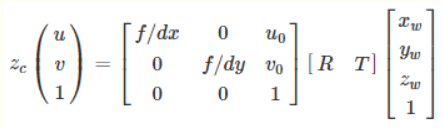其中u,v为二维平面上的任意点坐标，1为深度，Zc就是咱们的二维平面上的点。

其中R,T是外参矩阵，并且我们的世界坐标原点就是相机的坐标原点
所以，R，T可以取下面的矩阵，（具体原理的话比较复杂，我也不是很懂为什么会这样，我回头再补补数学）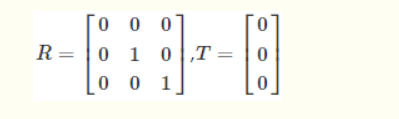带入得到：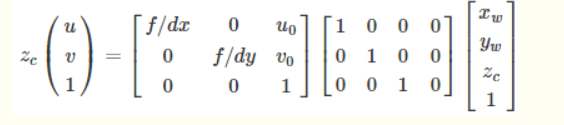最后转换得到这个公式：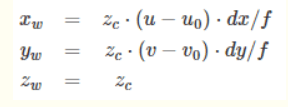那么接下来咱们就对这个公式进行处理，来生成我们的点云

# 数据准备

前面通过公式我们可以了解到，一个二维图像，具备了RGB信息，还有对应的那个像素点在二维空间的“投影”。我们需要将这个点重新还原到三维空间。所以这里需要使用到深度信息，而这个是需要一些专业摄像机才有的，比如iphone的，或者机器人的：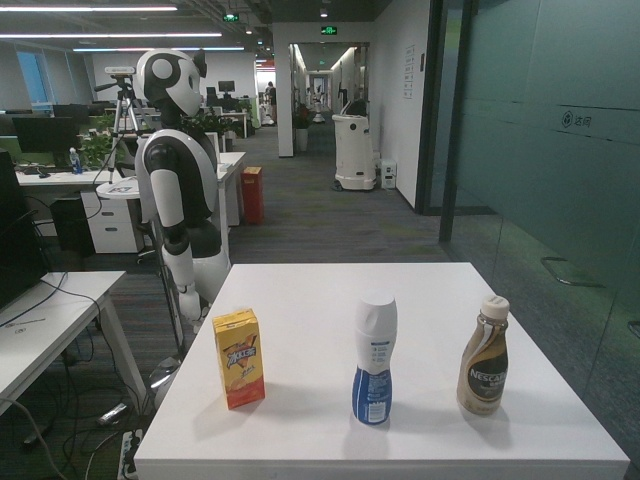有了这个深度图片，我们就可以还原坐标了。其实说到这儿，我们其实可以发现，RGB图片的话其实只是提供了一下色彩如果要还原为3D点云的话，因为深度图和对应的rgb的矩阵的位置是一一对应的。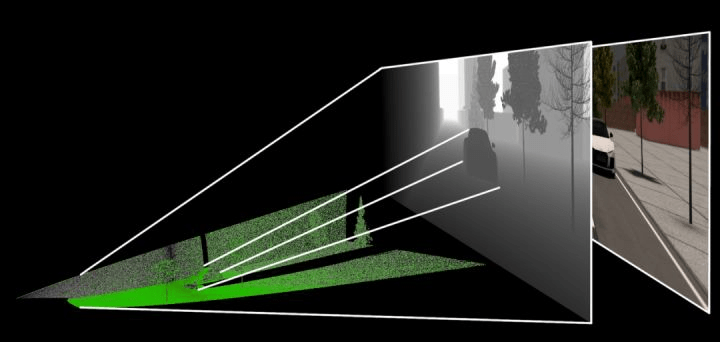# 图片加载

说到这里，我们就可以开始我们的正式编码了。

首先是咱们的图片加载阶段，这里的话主要是我们先需要一些信息，和读取图片。

``````
def __init__(self, rgb_file, depth_file, save_ply, camera_intrinsics=[784.0, 779.0, 649.0, 405.0]):
self.rgb_file = rgb_file
self.depth_file = depth_file
self.save_ply = save_ply

self.width = self.rgb.shape
self.height = self.rgb.shape

self.camera_intrinsics = camera_intrinsics
self.depth_scale = 1000

``````

下面的这个东西，就是咱们公式当中的u0,v0,dx,dy

``````camera_intrinsics=[784.0, 779.0, 649.0, 405.0]
``````

这个要注意的是，这个玩意呢，是不同的设备相机有不同的参数，要根据自己的设置。

一般来说相机会给这样的矩阵：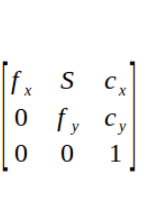我们对应起来就可以了。

之后是要注意的是这个深度图是uint16的，所以读取的时候加个-1这里还有个东西：
深度图与比例因子（scale_factor）
在代码中是：

``````self.depth_scale = 1000
``````

深度图对应的尺度因子是深度图中存储的值与真实深度（单位为m）的比例
通常情况下，深度值以毫米为单位存储在16位无符号整数中(0~65535)，因此要获得以米为单位的z值，深度图的像素需要除以比例因子1000。不过不同相机的的比例因子可能不同，我这里的话是1000，这个看自己的实际的。

# 算法实现

之后就是咱们的算法实现了，这个主要是位置换算，得到真实的x,y,z

``````
depth = np.asarray(self.depth, dtype=np.uint16).T
# depth[depth==65535]=0
self.Z = depth / self.depth_scale
fx, fy, cx, cy = self.camera_intrinsics

X = np.zeros((self.width, self.height))
Y = np.zeros((self.width, self.height))
for i in range(self.width):
X[i, :] = np.full(X.shape, i)

self.X = ((X - cx / 2) * self.Z) / fx
for i in range(self.height):
Y[:, i] = np.full(Y.shape, i)
self.Y = ((Y - cy / 2) * self.Z) / fy
``````

之后,x,y,z就算好了。

注意的的是我们计算完成后得到的x，y , x 的每一组向量都是 宽×高

# 生成点云

现在咱们已经得到了具体的坐标。那么接下来是要生成点云的，我们要把剩下的色彩信息搞上去。
代码很简单：

``````    data_ply = np.zeros((6, self.width * self.height))
data_ply = self.X.T.reshape(-1)
data_ply = -self.Y.T.reshape(-1)
data_ply = -self.Z.T.reshape(-1)
img = np.array(self.rgb, dtype=np.uint8)
data_ply = img[:, :, 0:1].reshape(-1)
data_ply = img[:, :, 1:2].reshape(-1)
data_ply = img[:, :, 2:3].reshape(-1)
self.data_ply = data_ply
t2 = time.time()
print('calcualte 3d point cloud Done.', t2 - t1)
``````

之后就是保存文件了。
目前点云的主要存储格式包括：pts、LAS、PCD、.xyz 和. pcap 等

例如：
pts 点云文件格式是最简便的点云格式，直接按 XYZ 顺序存储点云数据， 可以是整型或者浮点型。LAS 是激光雷达数据（LiDAR），存储格式比 pts 复杂，旨在提供一种开放的格式标准，允许不同的硬件和软件提供商输出可互操作的统一格式。LAS 格式点云截图，其中 C：class(所属类)，F：flight(航线号)，T：time(GPS 时间)，I：intensity(回波强度)，R：return(第几次回波)，N：number of return(回波次数)，A：scan angle(扫描角)，RGB：red green blue(RGB 颜色值)。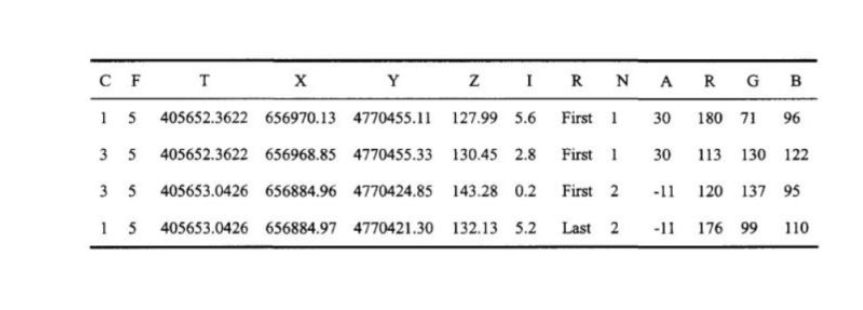等等，我们这里的是ply
全名为多边形档案（Polygon File Format）或 史丹佛三角形档案（Stanford Triangle Format）。. 该格式主要用以储存立体扫描结果的三维数值，透过多边形片面的集合描述三维物体，与其他格式相较之下这是较为简单的方法。. 它可以储存的资讯包含颜色、透明度、表面法向量、材质座标与资料可信度，并能对多边形的正反两面设定不同的属性。

格式为

头部

顶点列表

面片列表

其他元素列表``````       	start = time.time()
float_formatter = lambda x: "%.4f" % x
points = []
for i in self.data_ply.T:
points.append("{} {} {} {} {} {} 0\n".format
(float_formatter(i), float_formatter(i), float_formatter(i),
int(i), int(i), int(i)))

file = open(self.save_ply, "w")
file.write('''ply
format ascii 1.0
element vertex %d
property float x
property float y
property float z
property uchar red
property uchar green
property uchar blue
property uchar alpha
%s
''' % (len(points), "".join(points)))
file.close()

end = time.time()
print("Write into .ply file Done.", end - start)
``````

# 点云显示

这个的话可以使用软件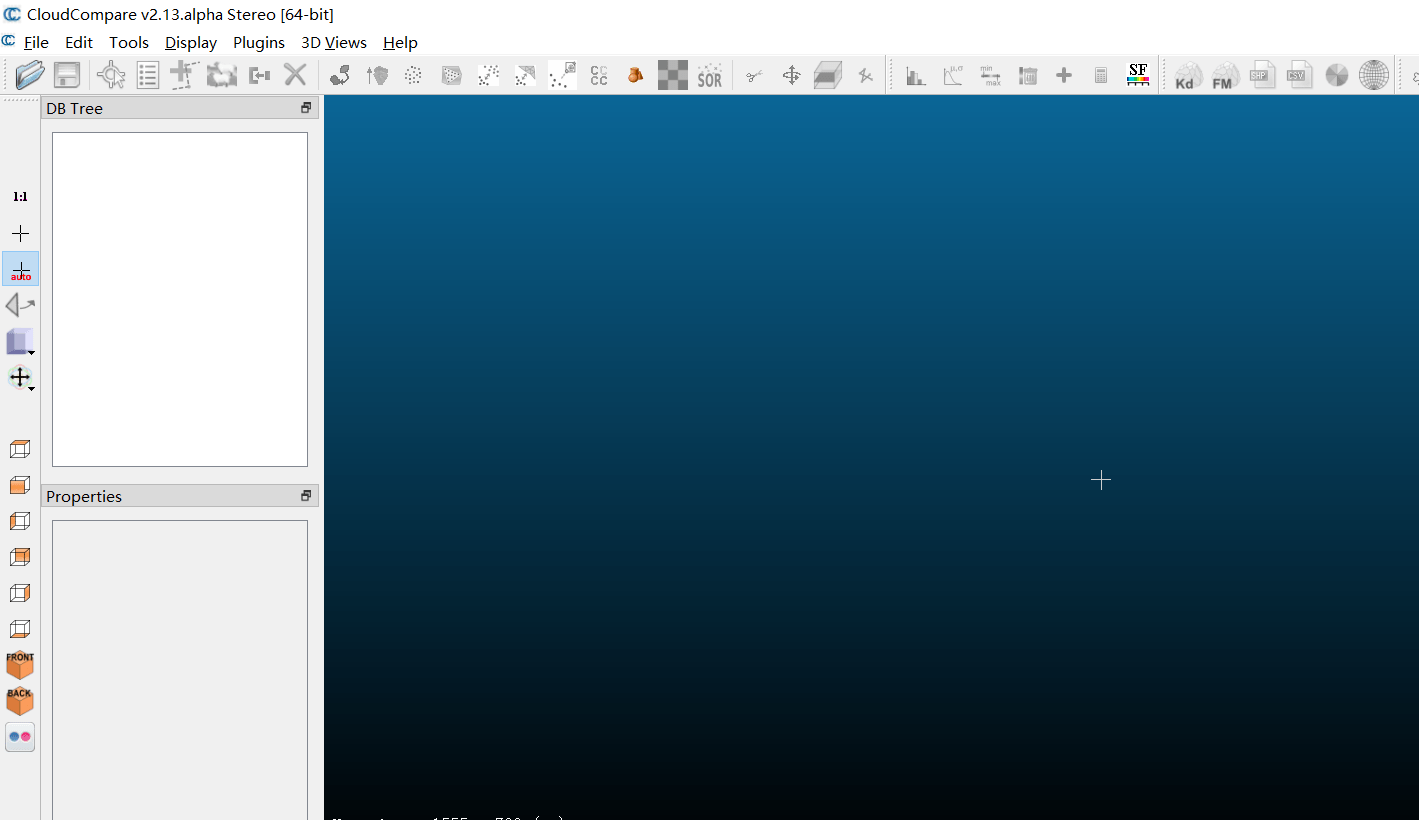也可以使用open3d模块

# 完整代码

``````import cv2
import numpy as np
import open3d as o3d

import time

class point_cloud_generator():

def __init__(self, rgb_file, depth_file, save_ply, camera_intrinsics=[784.0, 779.0, 649.0, 405.0]):
self.rgb_file = rgb_file
self.depth_file = depth_file
self.save_ply = save_ply

self.width = self.rgb.shape
self.height = self.rgb.shape

self.camera_intrinsics = camera_intrinsics
self.depth_scale = 1000

def compute(self):
t1 = time.time()

depth = np.asarray(self.depth, dtype=np.uint16).T
# depth[depth==65535]=0
self.Z = depth / self.depth_scale
fx, fy, cx, cy = self.camera_intrinsics

X = np.zeros((self.width, self.height))
Y = np.zeros((self.width, self.height))
for i in range(self.width):
X[i, :] = np.full(X.shape, i)

self.X = ((X - cx / 2) * self.Z) / fx
for i in range(self.height):
Y[:, i] = np.full(Y.shape, i)
self.Y = ((Y - cy / 2) * self.Z) / fy

data_ply = np.zeros((6, self.width * self.height))
data_ply = self.X.T.reshape(-1)
data_ply = -self.Y.T.reshape(-1)
data_ply = -self.Z.T.reshape(-1)
img = np.array(self.rgb, dtype=np.uint8)
data_ply = img[:, :, 0:1].reshape(-1)
data_ply = img[:, :, 1:2].reshape(-1)
data_ply = img[:, :, 2:3].reshape(-1)
self.data_ply = data_ply
t2 = time.time()
print('calcualte 3d point cloud Done.', t2 - t1)

def write_ply(self):
start = time.time()
float_formatter = lambda x: "%.4f" % x
points = []
for i in self.data_ply.T:
points.append("{} {} {} {} {} {} 0\n".format
(float_formatter(i), float_formatter(i), float_formatter(i),
int(i), int(i), int(i)))

file = open(self.save_ply, "w")
file.write('''ply
format ascii 1.0
element vertex %d
property float x
property float y
property float z
property uchar red
property uchar green
property uchar blue
property uchar alpha
%s
''' % (len(points), "".join(points)))
file.close()

end = time.time()
print("Write into .ply file Done.", end - start)

def show_point_cloud(self):
o3d.visualization.draw([pcd])

if __name__ == '__main__':
camera_intrinsics = [378.998657, 378.639862, 321.935120, 240.766663]
rgb_file = "data/1.jpg"
depth_file = "data/1.png"
save_ply = "data.ply"
a = point_cloud_generator(rgb_file=rgb_file,
depth_file=depth_file,
save_ply=save_ply,
camera_intrinsics=camera_intrinsics
)
a.compute()
a.write_ply()
a.show_point_cloud()

``````

效果如下：